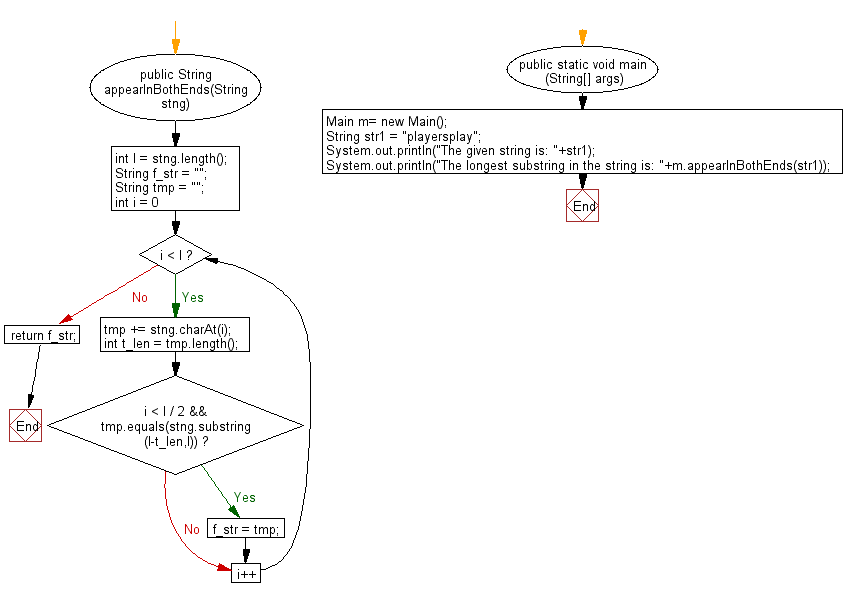﻿ Java exercises: Find the longest substring appears at both ends of a given string - w3resource# Java String Exercises: Find the longest substring appears at both ends of a given string

## Java String: Exercise-93 with Solution

Write a Java program to find the longest substring appears at both ends of a given string.

Sample Solution:

Java Code:

``````import java.util.*;
public class Main
{
public String appearInBothEnds(String stng)
{
int l = stng.length();
String f_str = "";
String tmp = "";
for (int i = 0; i < l; i++)
{
tmp += stng.charAt(i);
int t_len = tmp.length();
if (i < l / 2 && tmp.equals(stng.substring(l-t_len,l)))
f_str = tmp;
}
return f_str;
}

public static void main (String[] args)
{
Main m= new Main();
String str1 =  "playersplay";
System.out.println("The given string is: "+str1);
System.out.println("The longest substring in the string is: "+m.appearInBothEnds(str1));
}
}
``````

Sample Output:

```The given string is: playersplay
The longest substring in the string is: play
```

Pictorial Presentation:Flowchart:Java Code Editor:

s

Improve this sample solution and post your code through Disqus

What is the difficulty level of this exercise?

﻿